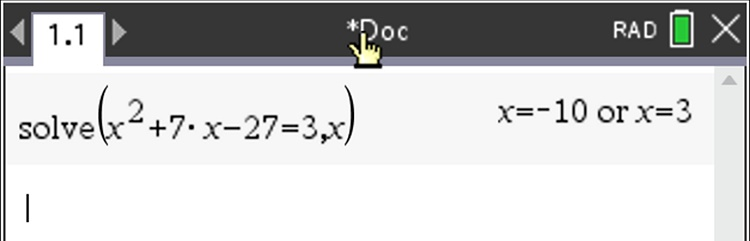# Communicating solutions where technology is used

When students are using technology for learning and doing mathematics, some procedures which might normally be performed using pen-and-paper will be performed using technology (Flynn & Asp, 2002).

In this case, students must make decisions about how they will communicate their mathematical solutions when the steps they might normally record using pen-and-paper are carried out using technology (Ball & Stacey, 2003).

## Understanding this strategy

To scaffold students to develop their ability to communicate solutions when they use technology, teachers can:

1. provide students with a problem to solve using pen-and-paper
2. model a pen-and-paper solution, calling on students to contribute to the steps recorded on the board
3. ask students to solve the same problem using technology
4. ask students to explain their strategies for solving using technology
5. model the use of technology as described by students
6. discuss what the solution to the given problem will be when technology is used.
7. ask students to compare and contrast their written solutions.

## Example using this strategy

The example below demonstrates this strategy in a Year 10 class solving quadratic equations (VCMNA337).

### Problem

Give students a quadratic equation to solve using pen-and-paper

Example
x2 + 7x - 27 = 3

### Modelling the solution on the board

Model the solution of the quadratic equation on the board:

• using factorisation and the null factor law

### Solving the equation

Two alternatives are shown below, using a computer algebra system (TI-Nspire):

1. Using the inbuilt 'solve' feature in one step2. Using factorisation, followed by 'solve'.

Note that some computer algebra systems use the term ‘factor’ to access the factorisation command, so students will need to be familiar with the syntax of their particular technology and be able to relate this to the mathematical language they might normally use.

### Scaffolding approaches

Scaffold students' use of a range of approaches by having some students explain their use of technology. Teacher questions could focus on approaches to use of technology, as well as syntax.

• What steps did you use to solve the problem?
• Did anyone use another method?

### Modelling the use of technology and discussion

Model the use of technology as students describe their approaches. Possible questions could include:

• What technology features are going to be useful here?
• What is the syntax for solving an equation?
• Where did you find the solve command? (i.e. helping students to articulate how they are navigating the technology).

Focus on communication of reasoning. For example, the use of words (i.e. 'solve', 'factor') to describe the procedures carried out using technology.

Written solutions can be compared and contrasted.